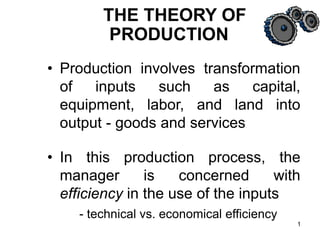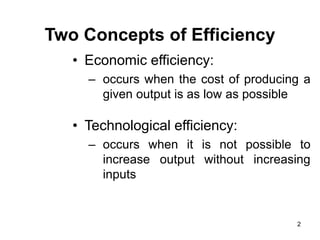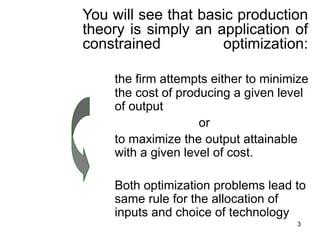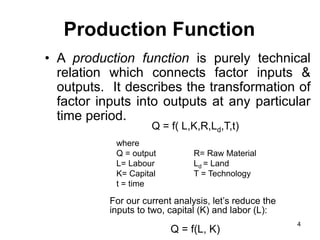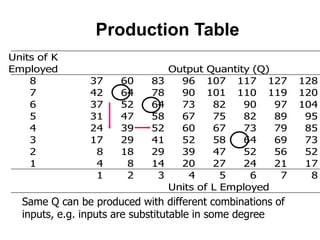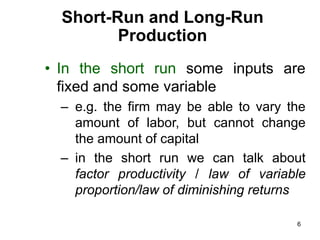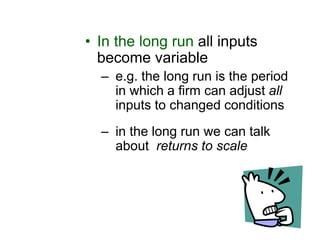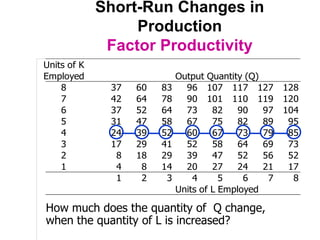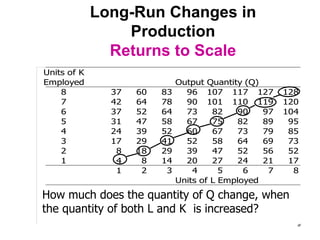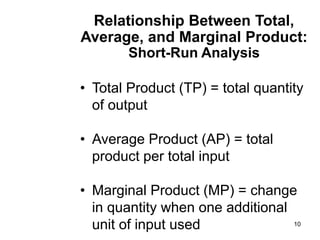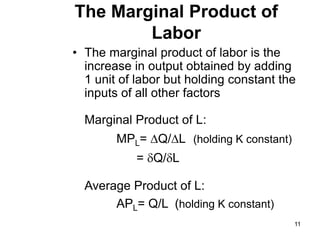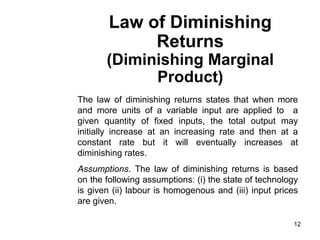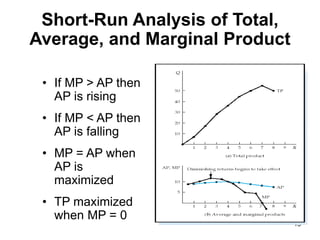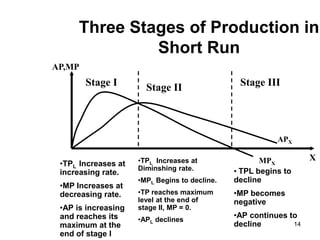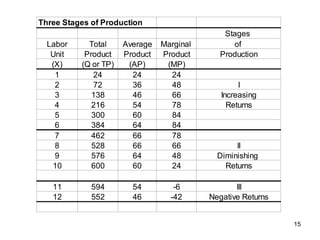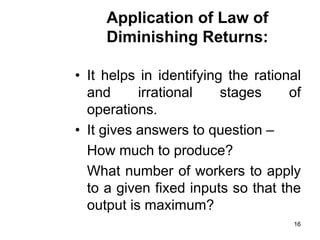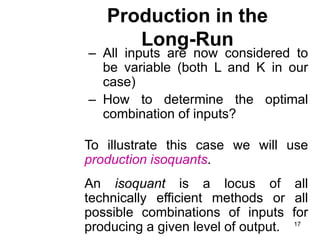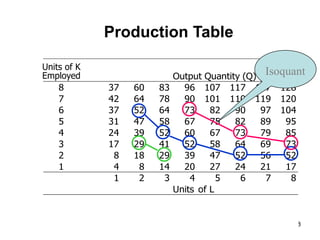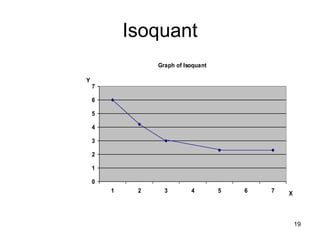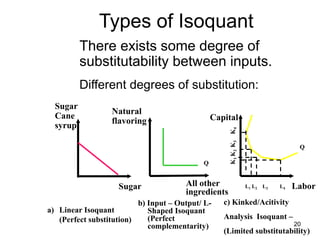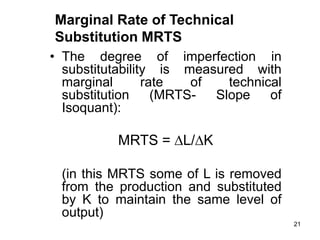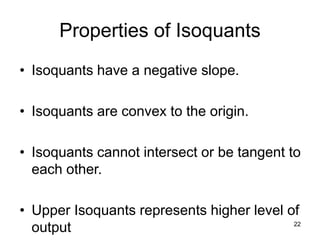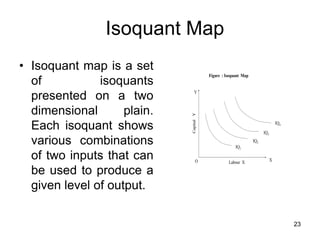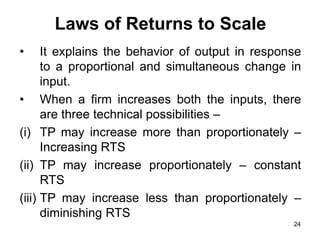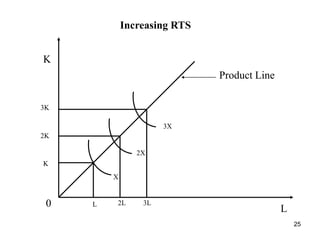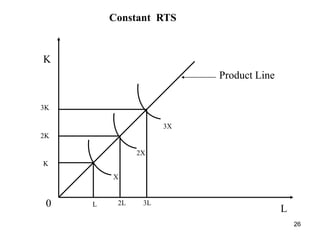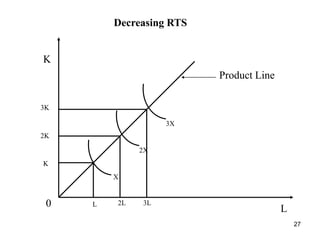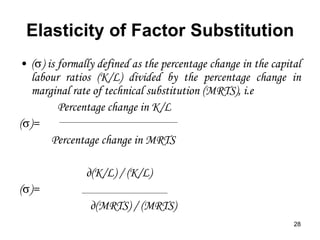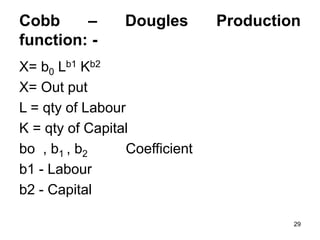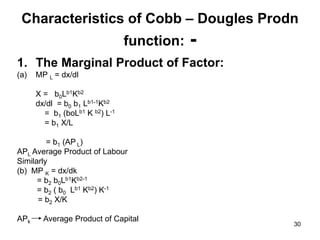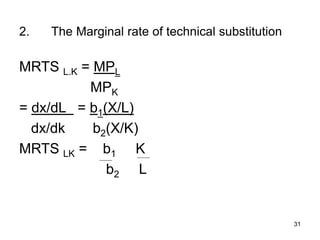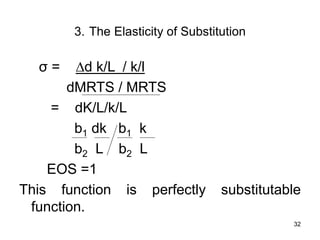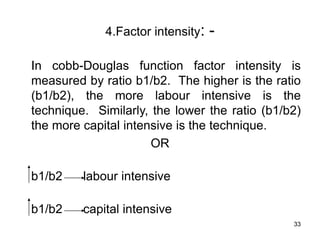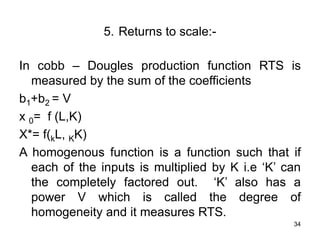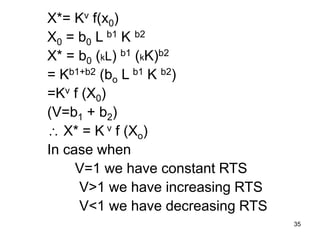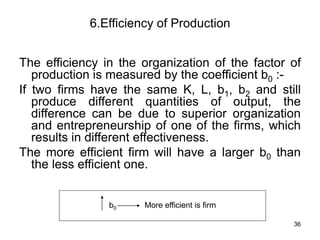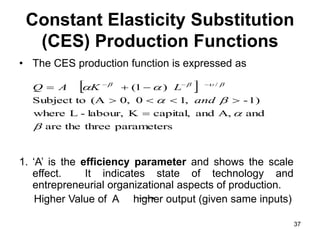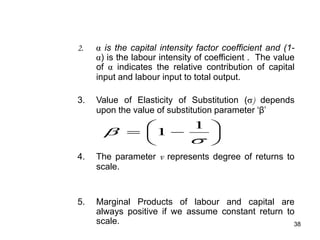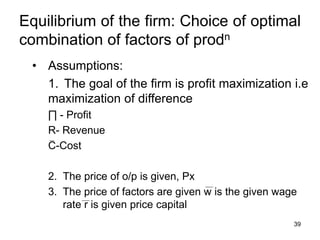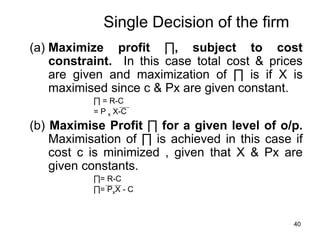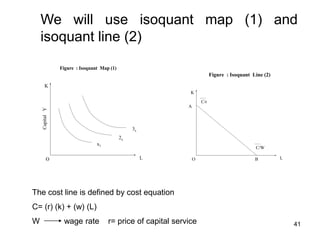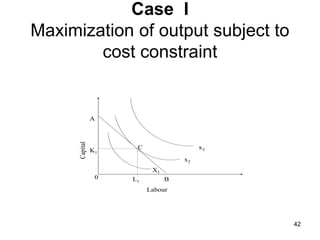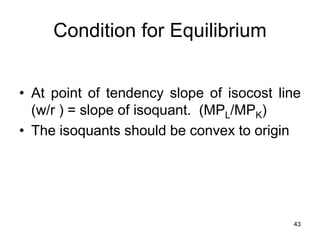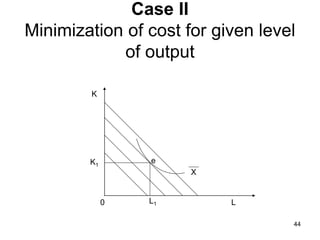1 of 44

### Production Slides (F).ppt

1. 1 • Production involves transformation of inputs such as capital, equipment, labor, and land into output - goods and services • In this production process, the manager is concerned with efficiency in the use of the inputs - technical vs. economical efficiency THE THEORY OF PRODUCTION
2. 2 Two Concepts of Efficiency • Economic efficiency: – occurs when the cost of producing a given output is as low as possible • Technological efficiency: – occurs when it is not possible to increase output without increasing inputs
3. 3 You will see that basic production theory is simply an application of constrained optimization: the firm attempts either to minimize the cost of producing a given level of output or to maximize the output attainable with a given level of cost. Both optimization problems lead to same rule for the allocation of inputs and choice of technology
4. 4 Production Function • A production function is purely technical relation which connects factor inputs & outputs. It describes the transformation of factor inputs into outputs at any particular time period. Q = f( L,K,R,Ld,T,t) where Q = output R= Raw Material L= Labour Ld = Land K= Capital T = Technology t = time For our current analysis, let’s reduce the inputs to two, capital (K) and labor (L): Q = f(L, K)
5. 5 Production Table Units of K Employed Output Quantity (Q) 8 37 60 83 96 107 117 127 128 7 42 64 78 90 101 110 119 120 6 37 52 64 73 82 90 97 104 5 31 47 58 67 75 82 89 95 4 24 39 52 60 67 73 79 85 3 17 29 41 52 58 64 69 73 2 8 18 29 39 47 52 56 52 1 4 8 14 20 27 24 21 17 1 2 3 4 5 6 7 8 Units of L Employed Same Q can be produced with different combinations of inputs, e.g. inputs are substitutable in some degree
6. 6 Short-Run and Long-Run Production • In the short run some inputs are fixed and some variable – e.g. the firm may be able to vary the amount of labor, but cannot change the amount of capital – in the short run we can talk about factor productivity / law of variable proportion/law of diminishing returns
7. 7 • In the long run all inputs become variable – e.g. the long run is the period in which a firm can adjust all inputs to changed conditions – in the long run we can talk about returns to scale
8. 8 Short-Run Changes in Production Factor Productivity Units of K Employed Output Quantity (Q) 8 37 60 83 96 107 117 127 128 7 42 64 78 90 101 110 119 120 6 37 52 64 73 82 90 97 104 5 31 47 58 67 75 82 89 95 4 24 39 52 60 67 73 79 85 3 17 29 41 52 58 64 69 73 2 8 18 29 39 47 52 56 52 1 4 8 14 20 27 24 21 17 1 2 3 4 5 6 7 8 Units of L Employed How much does the quantity of Q change, when the quantity of L is increased?
9. 9 Long-Run Changes in Production Returns to Scale Units of K Employed Output Quantity (Q) 8 37 60 83 96 107 117 127 128 7 42 64 78 90 101 110 119 120 6 37 52 64 73 82 90 97 104 5 31 47 58 67 75 82 89 95 4 24 39 52 60 67 73 79 85 3 17 29 41 52 58 64 69 73 2 8 18 29 39 47 52 56 52 1 4 8 14 20 27 24 21 17 1 2 3 4 5 6 7 8 Units of L Employed How much does the quantity of Q change, when the quantity of both L and K is increased?
10. 10 Relationship Between Total, Average, and Marginal Product: Short-Run Analysis • Total Product (TP) = total quantity of output • Average Product (AP) = total product per total input • Marginal Product (MP) = change in quantity when one additional unit of input used
11. 11 The Marginal Product of Labor • The marginal product of labor is the increase in output obtained by adding 1 unit of labor but holding constant the inputs of all other factors Marginal Product of L: MPL= Q/L (holding K constant) = Q/L Average Product of L: APL= Q/L (holding K constant)
12. 12 Law of Diminishing Returns (Diminishing Marginal Product) The law of diminishing returns states that when more and more units of a variable input are applied to a given quantity of fixed inputs, the total output may initially increase at an increasing rate and then at a constant rate but it will eventually increases at diminishing rates. Assumptions. The law of diminishing returns is based on the following assumptions: (i) the state of technology is given (ii) labour is homogenous and (iii) input prices are given.
13. 13 Short-Run Analysis of Total, Average, and Marginal Product • If MP > AP then AP is rising • If MP < AP then AP is falling • MP = AP when AP is maximized • TP maximized when MP = 0
14. 14 Three Stages of Production in Short Run AP,MP X Stage I Stage II Stage III APX MPX •TPL Increases at increasing rate. •MP Increases at decreasing rate. •AP is increasing and reaches its maximum at the end of stage I •TPL Increases at Diminshing rate. •MPL Begins to decline. •TP reaches maximum level at the end of stage II, MP = 0. •APL declines • TPL begins to decline •MP becomes negative •AP continues to decline
15. 15 Three Stages of Production Stages Labor Total Average Marginal of Unit Product Product Product Production (X) (Q or TP) (AP) (MP) 1 24 24 24 2 72 36 48 I 3 138 46 66 Increasing 4 216 54 78 Returns 5 300 60 84 6 384 64 84 7 462 66 78 8 528 66 66 II 9 576 64 48 Diminishing 10 600 60 24 Returns 11 594 54 -6 III 12 552 46 -42 Negative Returns
16. 16 Application of Law of Diminishing Returns: • It helps in identifying the rational and irrational stages of operations. • It gives answers to question – How much to produce? What number of workers to apply to a given fixed inputs so that the output is maximum?
17. 17 Production in the Long-Run – All inputs are now considered to be variable (both L and K in our case) – How to determine the optimal combination of inputs? To illustrate this case we will use production isoquants. An isoquant is a locus of all technically efficient methods or all possible combinations of inputs for producing a given level of output.
18. 18 Production Table Units of K Employed Output Quantity (Q) 8 37 60 83 96 107 117 127 128 7 42 64 78 90 101 110 119 120 6 37 52 64 73 82 90 97 104 5 31 47 58 67 75 82 89 95 4 24 39 52 60 67 73 79 85 3 17 29 41 52 58 64 69 73 2 8 18 29 39 47 52 56 52 1 4 8 14 20 27 24 21 17 1 2 3 4 5 6 7 8 Units of K Employed of L Isoquant Units of K Employed
19. 19 Isoquant Graph of Isoquant 0 1 2 3 4 5 6 7 1 2 3 4 5 6 7 X Y
20. 20 There exists some degree of substitutability between inputs. Different degrees of substitution: Sugar a) Linear Isoquant (Perfect substitution) b) Input – Output/ L- Shaped Isoquant (Perfect complementarity) All other ingredients Natural flavoring Q Q Capital Labor L1 L2 L3 L4 K 1 K 2 K 3 K 4 Sugar Cane syrup c) Kinked/Acitivity Analysis Isoquant – (Limited substitutability) Types of Isoquant
21. 21 • The degree of imperfection in substitutability is measured with marginal rate of technical substitution (MRTS- Slope of Isoquant): MRTS = L/K (in this MRTS some of L is removed from the production and substituted by K to maintain the same level of output) Marginal Rate of Technical Substitution MRTS
22. 22 Properties of Isoquants • Isoquants have a negative slope. • Isoquants are convex to the origin. • Isoquants cannot intersect or be tangent to each other. • Upper Isoquants represents higher level of output
23. 23 Isoquant Map • Isoquant map is a set of isoquants presented on a two dimensional plain. Each isoquant shows various combinations of two inputs that can be used to produce a given level of output. Figure : Isoquant Map Labour X Capital Y Y O X IQ4 IQ3 IQ2 IQ1
24. 24 Laws of Returns to Scale • It explains the behavior of output in response to a proportional and simultaneous change in input. • When a firm increases both the inputs, there are three technical possibilities – (i) TP may increase more than proportionately – Increasing RTS (ii) TP may increase proportionately – constant RTS (iii) TP may increase less than proportionately – diminishing RTS
25. 25 K 3K 2K K 3X 2X X L 0 L 2L 3L Increasing RTS Product Line
26. 26 K 3K 2K K 3X 2X X L 0 L 2L 3L Constant RTS Product Line
27. 27 K 3K 2K K 3X 2X X L 0 L 2L 3L Decreasing RTS Product Line
28. 28 Elasticity of Factor Substitution • ( ) is formally defined as the percentage change in the capital labour ratios (K/L) divided by the percentage change in marginal rate of technical substitution (MRTS), i.e Percentage change in K/L ( )= Percentage change in MRTS д(K/L) / (K/L) ( )= д(MRTS) / (MRTS)
29. 29 Cobb – Dougles Production function: - X= b0 Lb1 Kb2 X= Out put L = qty of Labour K = qty of Capital bo , b1 , b2 Coefficient b1 - Labour b2 - Capital
30. 30 Characteristics of Cobb – Dougles Prodn function: - 1. The Marginal Product of Factor: (a) MP L = dx/dl X = b0Lb1Kb2 dx/dl = b0 b1 Lb1-1Kb2 = b1 (boLb1 K b2) L-1 = b1 X/L = b1 (AP L) APL Average Product of Labour Similarly (b) MP K = dx/dk = b2 b0Lb1Kb2-1 = b2 ( b0 Lb1 Kb2) K-1 = b2 X/K APk Average Product of Capital
31. 31 2. The Marginal rate of technical substitution MRTS L.K = MPL MPK = dx/dL = b1(X/L) dx/dk b2(X/K) MRTS LK = b1 K b2 L
32. 32 3. The Elasticity of Substitution σ = d k/L / k/l dMRTS / MRTS = dK/L/k/L b1 dk b1 k b2 L b2 L EOS =1 This function is perfectly substitutable function.
33. 33 4.Factor intensity: - In cobb-Douglas function factor intensity is measured by ratio b1/b2. The higher is the ratio (b1/b2), the more labour intensive is the technique. Similarly, the lower the ratio (b1/b2) the more capital intensive is the technique. OR b1/b2 labour intensive b1/b2 capital intensive
34. 34 5. Returns to scale:- In cobb – Dougles production function RTS is measured by the sum of the coefficients b1+b2 = V x 0= f (L,K) X*= f(kL, KK) A homogenous function is a function such that if each of the inputs is multiplied by K i.e ‘K’ can the completely factored out. ‘K’ also has a power V which is called the degree of homogeneity and it measures RTS.
35. 35 X*= Kv f(x0) X0 = b0 L b1 K b2 X* = b0 (kL) b1 (kK)b2 = Kb1+b2 (bo L b1 K b2) =Kv f (X0) (V=b1 + b2)  X* = K v f (Xo) In case when V=1 we have constant RTS V>1 we have increasing RTS V<1 we have decreasing RTS
36. 36 6.Efficiency of Production The efficiency in the organization of the factor of production is measured by the coefficient b0 :- If two firms have the same K, L, b1, b2 and still produce different quantities of output, the difference can be due to superior organization and entrepreneurship of one of the firms, which results in different effectiveness. The more efficient firm will have a larger b0 than the less efficient one. b0 More efficient is firm
37. 37 Constant Elasticity Substitution (CES) Production Functions • The CES production function is expressed as 1. ‘A’ is the efficiency parameter and shows the scale effect. It indicates state of technology and entrepreneurial organizational aspects of production. Higher Value of A higher output (given same inputs)   parameters three the are and A, and capital, K labour, - L where -1) , 1 0 , 0 (A Subject to ) 1 ( /                      and L K A Q
38. 38 2. is the capital intensity factor coefficient and (1- ) is the labour intensity of coefficient . The value of indicates the relative contribution of capital input and labour input to total output. 3. Value of Elasticity of Substitution ( ) depends upon the value of substitution parameter ‘β’ 4. The parameter v represents degree of returns to scale. 5. Marginal Products of labour and capital are always positive if we assume constant return to scale.           1 1
39. 39 Equilibrium of the firm: Choice of optimal combination of factors of prodn • Assumptions: 1. The goal of the firm is profit maximization i.e maximization of difference ∏ - Profit R- Revenue C-Cost 2. The price of o/p is given, Px 3. The price of factors are given w is the given wage rate r is given price capital
40. 40 Single Decision of the firm (a) Maximize profit ∏, subject to cost constraint. In this case total cost & prices are given and maximization of ∏ is if X is maximised since c & Px are given constant. ∏ = R-C = P x X-C (b) Maximise Profit ∏ for a given level of o/p. Maximisation of ∏ is achieved in this case if cost c is minimized , given that X & Px are given constants. ∏= R-C ∏= PxX - C
41. 41 We will use isoquant map (1) and isoquant line (2) Figure : Isoquant Line (2) K O L C/W C/r B A Figure : Isoquant Map (1) Capital Y K O L 3x 2x x1 The cost line is defined by cost equation C= (r) (k) + (w) (L) W wage rate r= price of capital service
42. 42 Case I Maximization of output subject to cost constraint Labour 0 L1 K1 C x3 x2 X1 A B Capital
43. 43 Condition for Equilibrium • At point of tendency slope of isocost line (w/r ) = slope of isoquant. (MPL/MPK) • The isoquants should be convex to origin
44. 44 Case II Minimization of cost for given level of output e K 0 L1 K1 L X